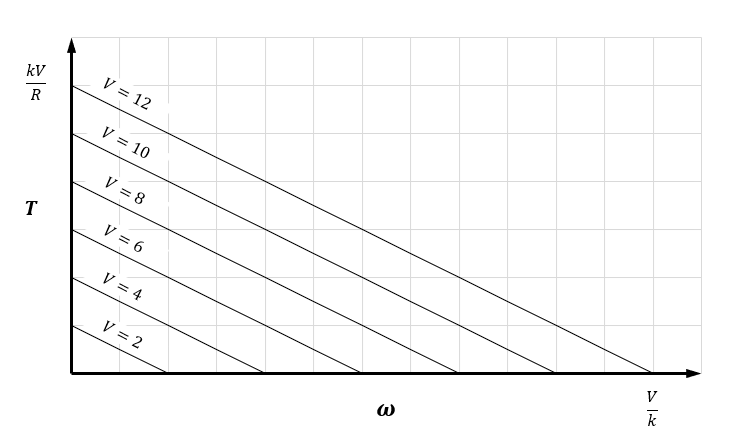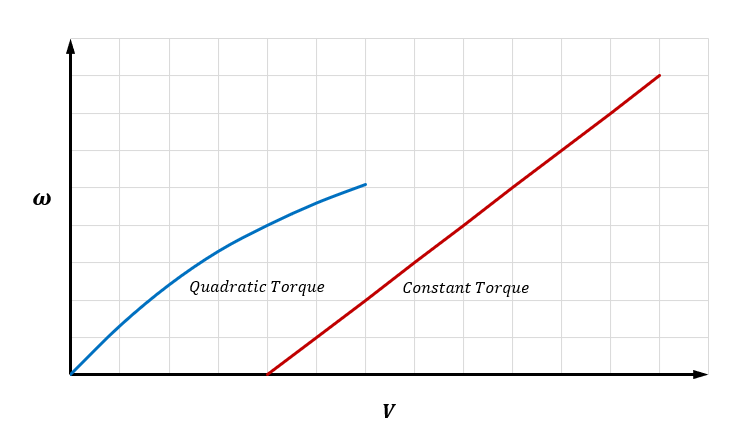# DC MotorsWritten by

At some point in time, we all need to put our electronics to work to actually do something in the physical world. For this we need actuators of some kind—devices that turn electrical signals into physical movement. One of the most common forms of actuators you are likely to encounter is the Permanent Magnet Brushed DC Motor. They are cheap, simple to drive with only two terminals and ubiquitous.

There are plenty of references which cover how they are constructed and how they work, so I’m going to focus on how to understand the way they turn voltage and current into torque and rotational speed (or angular velocity as it should be more properly called), and what that means for you as a circuit designer.FIGURE 1. The equivalent circuit of a brushed DC motor is quite simple. In most cases the inductance can be ignored because the electrical L/R time constant is usually much lower than the mechanical time constant given by the rotor inertia.

The equivalent circuit of a brushed permanent magnet DC motor is shown in Figure 1. Resistor R and inductor L represent the resistance and inductance seen at the terminals respectively. The voltage source E represents the back-emf generated by the motor windings as they rotate in the magnetic field. This voltage is the opposite sign to the terminal voltage and is proportional to the angular velocity of the motor as per the equation:

The torque the motor produces is proportional to the current that flows through the motor according to a similar equation:

The constants kTand ks are given in the motor’s data sheet. They may appear to be different depending on the units used, but if you translate them both to SI units in both cases, you will find they are the same number!

In analyzing the motor, we can usually ignore the inductance since theL/R electrical time constant is much shorter than the mechanical time constant determined by the rotor’s moment of inertia. This simplifies the analysis and we can see the motor current will be given by Ohm’s Law.

and therefore, the motor torque must be

Now we can construct a series of torque-speed curves for the DC motor at different terminal voltages. Figure 2 shows how this looks. The stall torque (when ωis zero) will bekV/R and the free-running speed (when T is zero) will be V/k.FIGURE 2. The torque-speed curves of a 12 V DC motor at various voltages are shown in this Figure. The Y-intercept gives the motor’s stall torque and the X-intercept gives the no-load speed (or angular velocity if we are being pedantic).

To understand what happens when a load is applied, we need to plot the load characteristics on the torque speed curve as we have done in Figure 3. A constant torque load (such as a traction load) is represented by the horizontal line and a quadratic load (such as a fan or centrifugal pump) is represented by the parabolic curve. The intersection between the motor and load torque-speed curve at a given voltage represents the operating point.FIGURE 3. To find the operating point, the load torque-speed characteristic of the load is superimposed on the motor curves.  The red and blue curves represent a constant torque load and a quadratic torque load respectively. The dots show the operating point at various motor voltages.

From this we can plot the motor speed as a function of voltage for a given load as shown in Figure 4. Here you can see that the constant torque load will not begin rotating until the voltage has reached a level to produce sufficient motor torque to overcome the load torque but will then increase in speed more or less linearly with voltage. On the other hand, the quadratic load will start rotating at a low voltage but will not increase in speed linearly.FIGURE 4. These curves show the information in Figure 3 in another way, relating the motor speed to terminal voltage for each type of load. The constant torque load won’t start until the motor torque is enough to overcome the load. The quadratic load starts at low speed, but the curve is not linear.

How do we make sense of all this? The key is to understand the torque speed characteristics of your load and how it interacts with the motor. This allows you to get a sense of how the speed will vary with motor voltage. If you need precision speed regulation you will need to resort to closed loop techniques using a speed sensor of some kind.

References:

“Brushed DC Motors from Maxon | Maxon Group.” Accessed November 27, 2020. https://www.maxongroup.net.au/maxon/view/content/overview-dc-motor.

“D.C. Motor Torque/Speed Curve Tutorial: Understanding Motor Characteristics.” Accessed November 27, 2020. http://lancet.mit.edu/motors/motors3.html

 Keep up-to-date with our FREE Weekly Newsletter! Don't miss out on upcoming issues of Circuit Cellar.— ADVERTISMENT— —Advertise Here— Subscribe to Circuit Cellar Magazine Note: We’ve made the May 2020 issue of Circuit Cellar available as a free sample issue. In it, you’ll find a rich variety of the kinds of articles and information that exemplify a typical issue of the current magazine. Would you like to write for Circuit Cellar? We are always accepting articles/posts from the technical community. Get in touch with us and let's discuss your ideas.